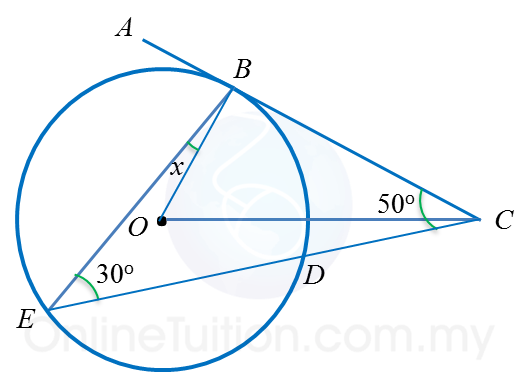# 6.3.2 Tangents to a Circle (Sample Questions)

Example 1:In the diagram, is the centre of a circle. ABC and CDE are two tangents to the circle at points B and D respectively. Find the length of OC.

Solution:
OC2= OB2 + BC2(Pythagoras’ Theorem)
= 62 + 82
= 100
OC = √100 = 10 cm

Example 2:In the diagram, AB and BC are two tangents to a circle with centre O. Calculate the values of
(a) x   (b) y

Solution:
(a)
AB = BC
7 + x = 12
x = 5

(b)
$\angle$ OBA = $\angle$ OBC = 21o
$\angle$ OAB = 90o(OA is perpendicular to AB)
yo= 180o – 21o – 90o
y = 69

Example 3:In the diagram, ABC is a tangent to the circle with centre Oat point B. CDE  is a straight line. Find the value of x.

Solution:
$\angle$ CBO = 90o ← (OB is perpendicular to BC)
In ∆ BCE,
=  180o – 30o – 50o– 90o
x =  10o